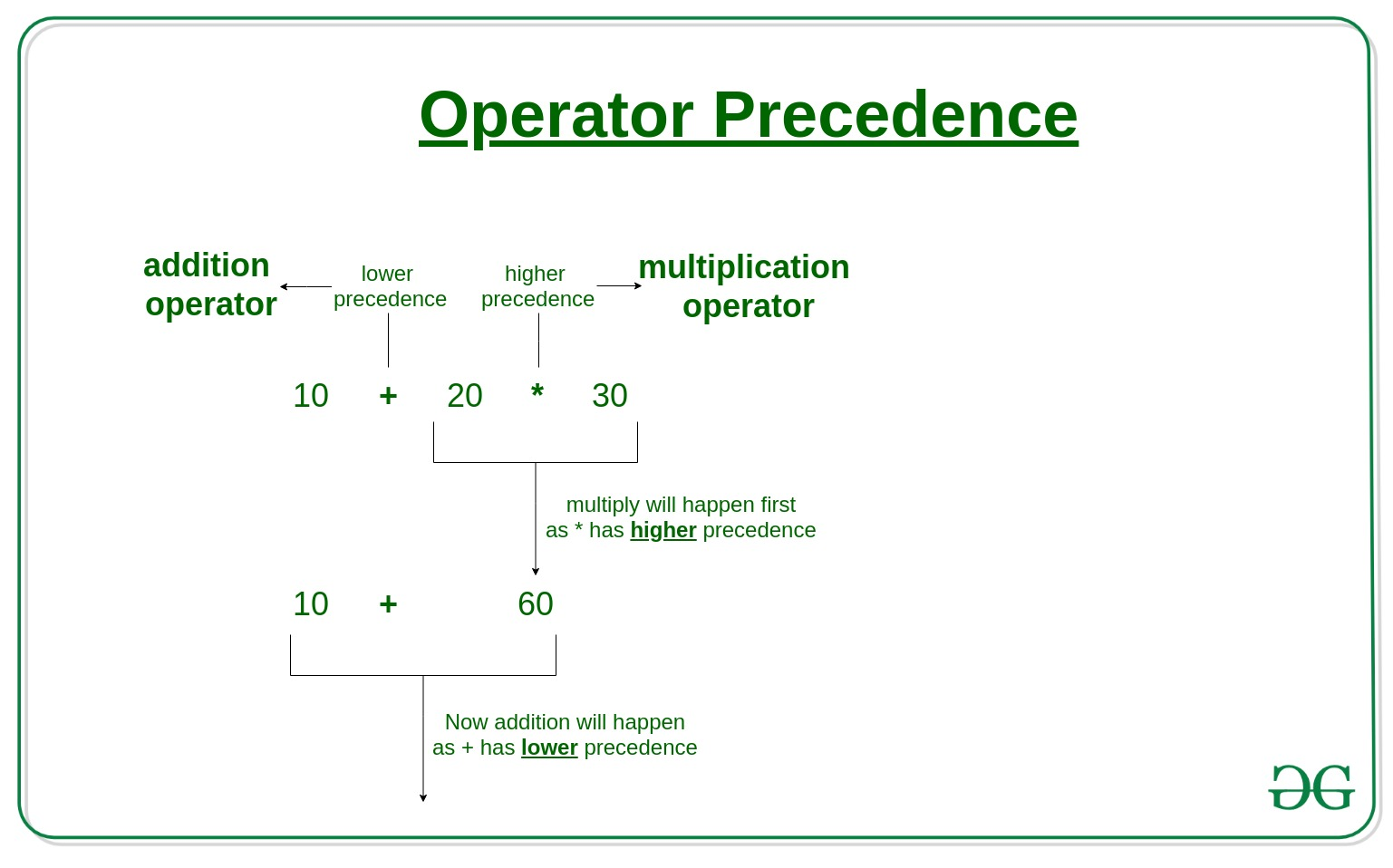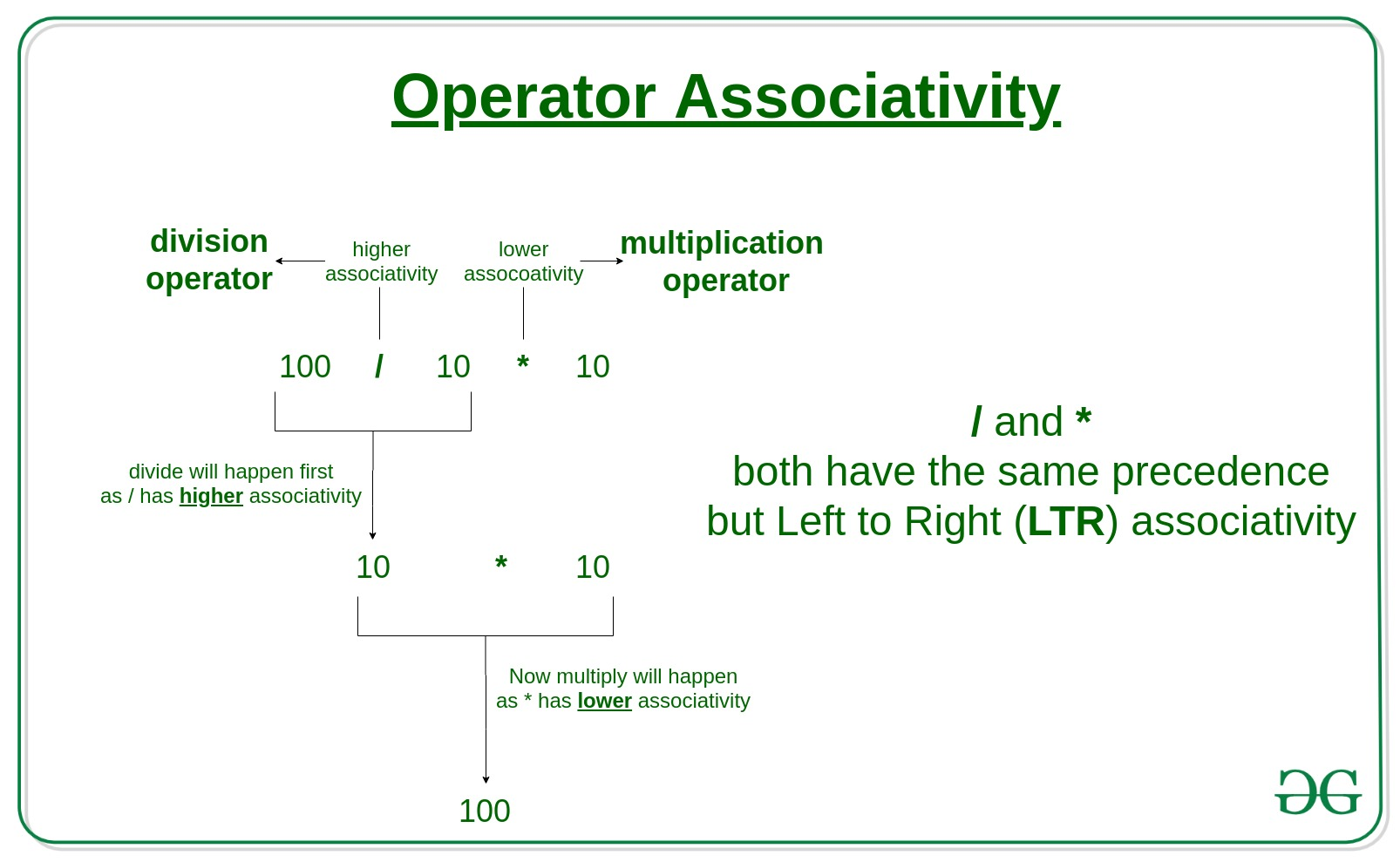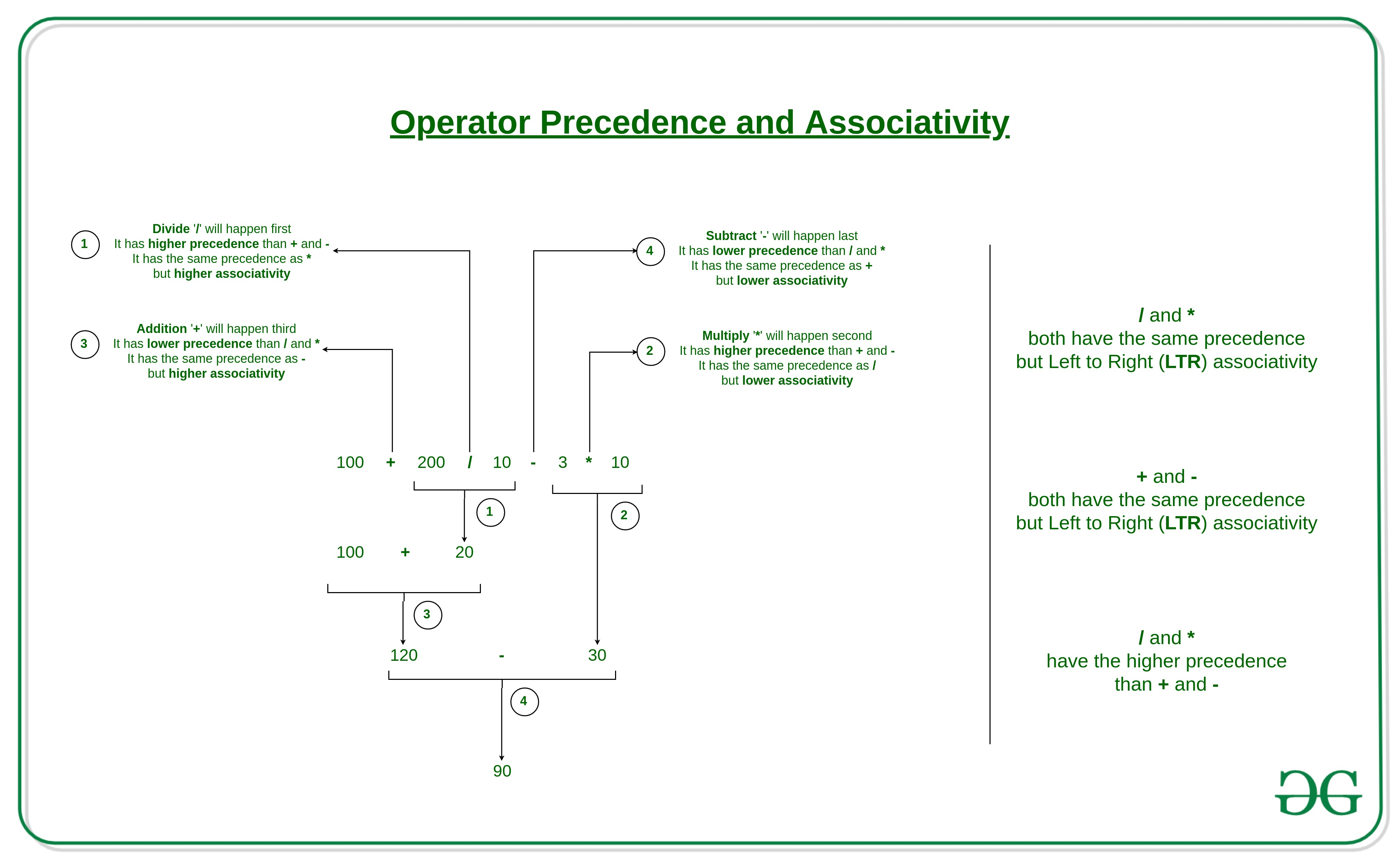Open in App
Not now

# Precedence and Associativity of Operators in Python

• Difficulty Level : Basic
• Last Updated : 29 Oct, 2021

When dealing with operators in Python we have to know about the concept of Python operator precedence and associativity as these determine the priorities of the operator otherwise, we’ll see unexpected outputs.

Operator Precedence: This is used in an expression with more than one operator with different precedence to determine which operation to perform first.

Example: Solve

```10 + 20 * 30
``````10 + 20 * 30 is calculated as 10 + (20 * 30)
and not as (10 + 20) * 30
```

Code:

## Python3

 `# Precedence of '+' & '*'``expr ``=` `10` `+` `20` `*` `30`` ` `print``(expr)`

Output:

```610
```

Example: Now, let’s see an example on logicalor‘ & logical and‘  operator.  ‘if‘ block is executed even if the age is 0. Because precedence of logical ‘and‘ is greater than the logical ‘or‘.

## Python3

 `# Precedence of 'or' & 'and'``name ``=` `"Alex"``age ``=` `0`` ` `if` `name ``=``=` `"Alex"` `or` `name ``=``=` `"John"` `and` `age >``=` `2` `:``    ``print``(``"Hello! Welcome."``)``else` `:``    ``print``(``"Good Bye!!"``)`

Output:

```Hello! Welcome.
```

Hence, To run the ‘else‘ block we can use parenthesis( ) as their precedence is highest among all the operators.

## Python3

 `# Precedence of 'or' & 'and'``name ``=` `"Alex"``age ``=` `0`` ` `if` `( name ``=``=` `"Alex"` `or` `name ``=``=` `"John"` `) ``and` `age >``=` `2` `:``  ``print``(``"Hello! Welcome."``)``else` `:``  ``print``(``"Good Bye!!"``)`

Output:

```Good Bye!!
```

Operator Associativity: If an expression contains two or more operators with the same precedence then Operator Associativity is used to determine. It can either be Left to Right or from Right to Left.

Example: ‘*’ and ‘/’ have the same precedence and their associativity is Left to Right, so the expression “100 / 10 * 10” is treated as “(100 / 10) * 10”.Code:

## Python3

 `# Left-right associativity``# 100 / 10 * 10 is calculated as ``# (100 / 10) * 10 and not ``# as 100 / (10 * 10)``print``(``100` `/` `10` `*` `10``)`` ` `# Left-right associativity``# 5 - 2 + 3 is calculated as ``# (5 - 2) + 3 and not ``# as 5 - (2 + 3)``print``(``5` `-` `2` `+` `3``)`` ` `# left-right associativity``print``(``5` `-` `(``2` `+` `3``))`` ` `# right-left associativity``# 2 ** 3 ** 2 is calculated as ``# 2 ** (3 ** 2) and not ``# as (2 ** 3) ** 2``print``(``2` `*``*` `3` `*``*` `2``)`

Output:

```100
6
0
512
```

Operators Precedence and Associativity are two main characteristics of operators that determine the evaluation order of sub-expressions in absence of brackets.

Example: Solve

```100 + 200 / 10 - 3 * 10
``````100 + 200 / 10 - 3 * 10 is calculated as 100 + (200 / 10) - (3 * 10)
and not as (100 + 200) / (10 - 3) * 10
```

Code:

## Python3

 `expression  ``=` `100` `+` `200` `/` `10` `-` `3` `*` `10``print``(expression )`

Output:

```90.0
```

Please see the following precedence and associativity table for reference. This table lists all operators from the highest precedence to the lowest precedence.

My Personal Notes arrow_drop_up